# Wikipedia Voting Network

## Examples

### Basic Examples

Retrieve the graph:

 In:=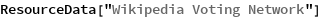Out=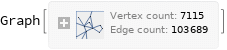Summary properties:

 In:=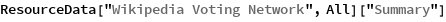Out=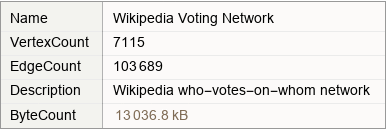### Basic Applications

Show the person with the most votes:

 In:=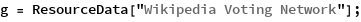In:=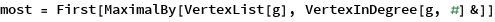Out=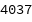In:=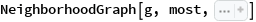Out=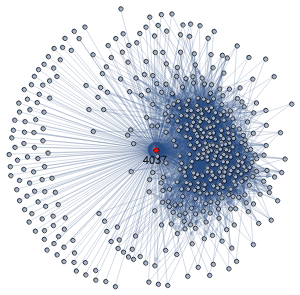Wolfram Research, "Wikipedia Voting Network" from the Wolfram Data Repository (2019)